###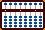ADDITION EXAMPLES

The Bead Unbaffled - An Abacus Manual

**All of the following examples will require using complementary numbers. In order to fully understand the technique, it's best to work these examples using your abacus.**

Example: 34 + 78 = 112`Step 1` ```A B C . 0 3 4 ``` ` `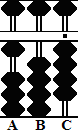``` Step 2 A B C . 0 3 4 -3 Step 2 0 0 4 +1    Step 2a 1 0 4```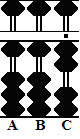```Step 3 A B C . 1 0 4 -2 Step 3 1 0 2 +1   Step 3a 1 1 2```

Step 1: Set 34 on rods BC.

Step 2: Add 7 to tens rod B. Subtract complement 3, then....
Step 2a: Carry 1 to hundreds rod A leaving 104 on rods ABC.

Step 3: Add 8 to units Rod C. Subtract the complement 2, then....
Step 3a and the answer: Carry 1 to tens rod B leaving 112 on rods ABC.

Note the decimal numbers in the following two examples. Rod B is the designated unit rod.

Example: 8.9 + 56 = 64.9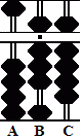```Step 1 A B C .   0 8 9 ``` ` `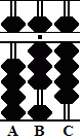``` Step 2 A B C .   0 8 9 +5 Step 2 5 8 9 ```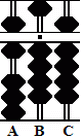```Step 3 A B C .    5 8 9 -4 Step 3 5 4 9 +1   Step 3a 6 4 9```

Step 1: Designate rod B as the unit rod. Set 8.9 on rods BC.

Step 2:  Add 5 to tens rod A leaving 58.9

Step 3: Add 6 to units rod B. Subtract the complement 4, then....
Step 3a and the answer: Carry1 to tens rod A leaving 64.9 on rods ABC.

This next example will be a little bit more difficult. With a little practice, solving problems of this type soon becomes second nature.

Example: 45.7 + 4.5 = 50.2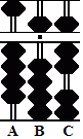```Step 1 A B C .   4 5 7 ``` ` ````Step 2 A B C .   4 5 7 +4   Step 2 4 9 7``` ` ```` Step 3 A B C .   4 9 7 -5 Step 3 4 9 2 -9   Step 3a 4 0 2 +1    Step 3b 5 0 2```

Step 1: Designate rod B as the unit rod. Set 45.7 on rods ABC.

Step 2: Add 4 to units rod B. This leaves 49.7 on rods ABC.

Step 3: Add 5 to tenths rod C. Use the complement. Subtract 5, then....
Step 3a: Carry 1 to units rod B. Rod B is full. Subtract the complement 9, then....
Step 3b and the answer: Carry 1 to tens rod A. This leaves 50.2 on rods ABC.

Print Page (.pdf format,96kb)